• 本次实验要求掌握触发器的基本原理，掌握寄存器和寄存器堆的组成原理。
•研究论文
• 1. 学习使用Verilog HDL语言进行时序电路的设计方法 2. 掌握灵活运用Verilog HDL语言进行行为级语言描述的技巧和方法; 3. 学习寄存器堆的数据传送和读写工作原理,掌握寄存器堆的设计方法.
• verilog hdl 设计寄存器堆，采用译码器、寄存器、数据选择等组合电路
•研究论文
• 这次要做的是用Verilog代码写一个寄存器堆，此寄存器堆共有32个寄存器，每个寄存器可存储32个二进制位。要求有一个写端口，两个读端口，本次实验设计为异步读同步写的寄存器堆，即读寄存器不需要时钟控制，但写...
这次要做的是用Verilog代码写一个寄存器堆，此寄存器堆共有32个寄存器，每个寄存器可存储32个二进制位。要求有一个写端口，两个读端口，本次实验设计为异步读同步写的寄存器堆，即读寄存器不需要时钟控制，但写寄存器需时钟控制。
先上寄存器堆模块的代码
timescale 1ns / 1ps
//*************************************************************************
//   > 文件名: regfile.v
//   > 描述  ：寄存器堆模块，同步写，异步读
//   > 作者  : LOONGSON
//   > 日期  : 2016-04-14
//*************************************************************************
module regfile(
input             clk,      //时钟控制信号
input             wen,      //写使能信号，1有效
input      [4 :0] raddr1,   //第一个读端口的地址
input      [4 :0] raddr2,   //第二个读端口的地址
input      [4 :0] waddr,    //一个写端口
input      [31:0] wdata,    //需要写入的数据
output  [31:0] rdata1,   //读出的数据1
output  [31:0] rdata2,   //读出的数据2
input      [4 :0] test_addr,    //输入的调试地址
output  [31:0] test_data     //输出调试数据
);
//总共32个寄存器
integer i = 0;
reg [31:0] REG_Files[31:0];
initial//初始化32个寄存器，全为0
for(i = 0;i < 32;i = i + 1)
REG_Files[i]<=0;
always @ (posedge clk)
begin
if(wen)
REG_Files[waddr] <= wdata;
end
assign rdata1 = REG_Files[raddr1] ;
assign rdata2 = REG_Files[raddr2];
assign test_data  = REG_Files[test_addr];
endmodule


其实整个代码的逻辑还是比较简单的。定义一个reg型数组REG_Files来充当寄存器堆，此数组共有32个元素，每一个元素的大小为32个二进制位。在initial块中，用for循环对寄存器堆的内容初始化为0。当时钟信号clk上跳沿时触发always语句的执行，如果写使能信号wen为1，则把数据写入寄存器堆中。因为是异步读，所以只要是输入寄存器的地址，应能够立刻得到寄存器的内容。用assign语句对读数据的输出端口rdata1和rdata2进行赋值，其中，数组的下标相当于寄存器的地址，因此可以写成REG_Files[raddr1] 这种形式。最后一个assign语句是上板验证时用到的，用test_data来向显示屏传送数据。如果仅仅是为了得到仿真图形，可以不用管这行代码，但如果想上板验证，可以查看我的另一篇博客中关于test_data的介绍。点击此处进行跳转
仿真图形
写寄存器阶段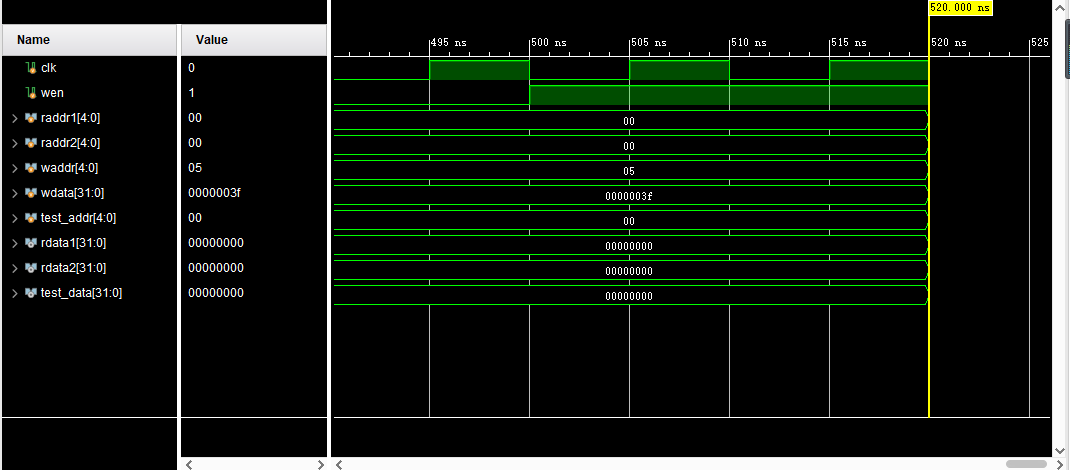读寄存器阶段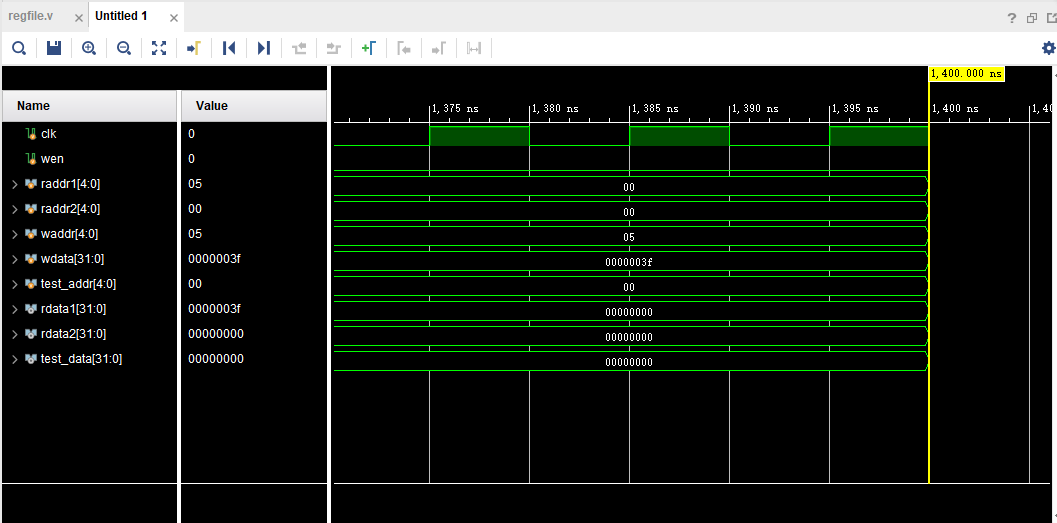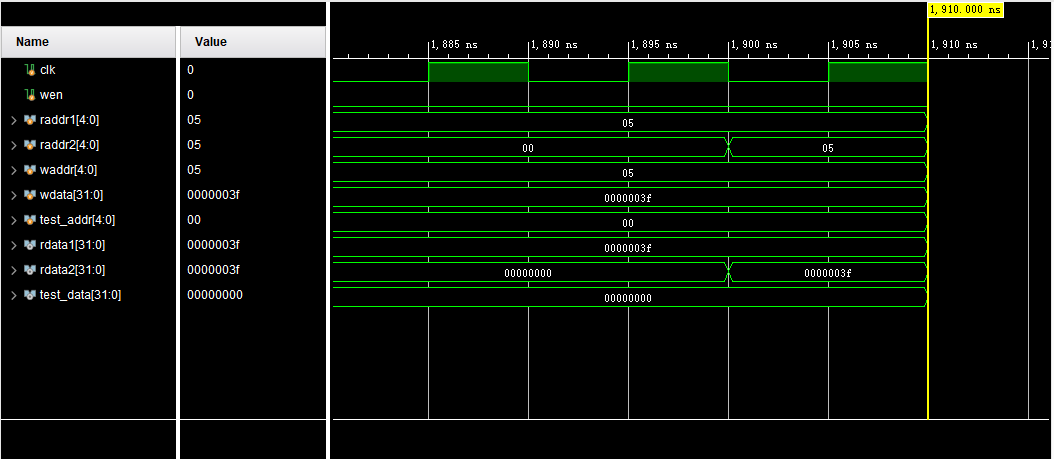上板验证图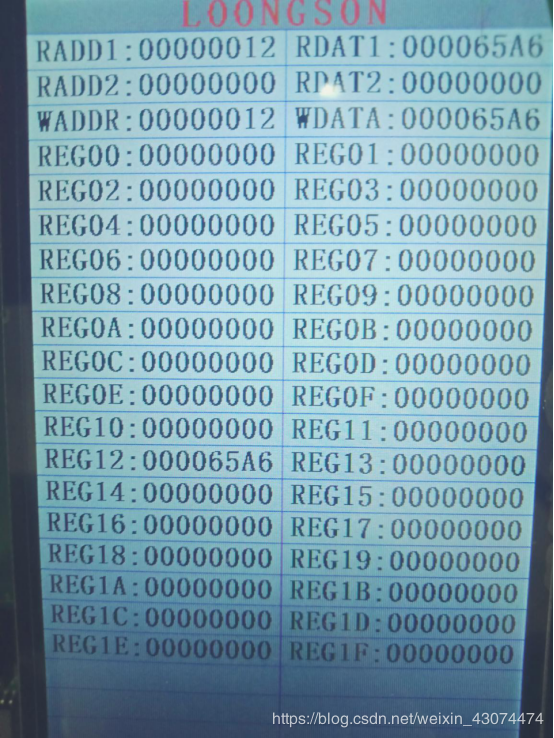regfile模块结构框图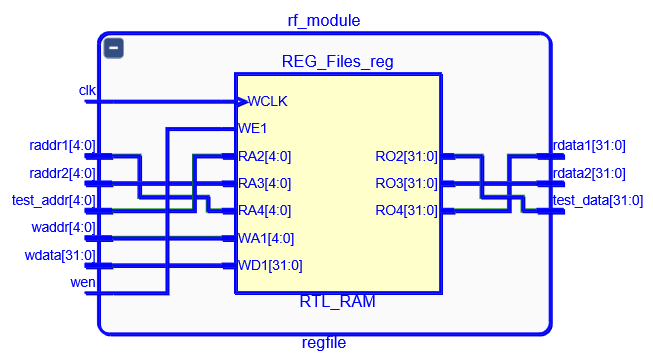寄存器堆设计实验的顶层模块大致框图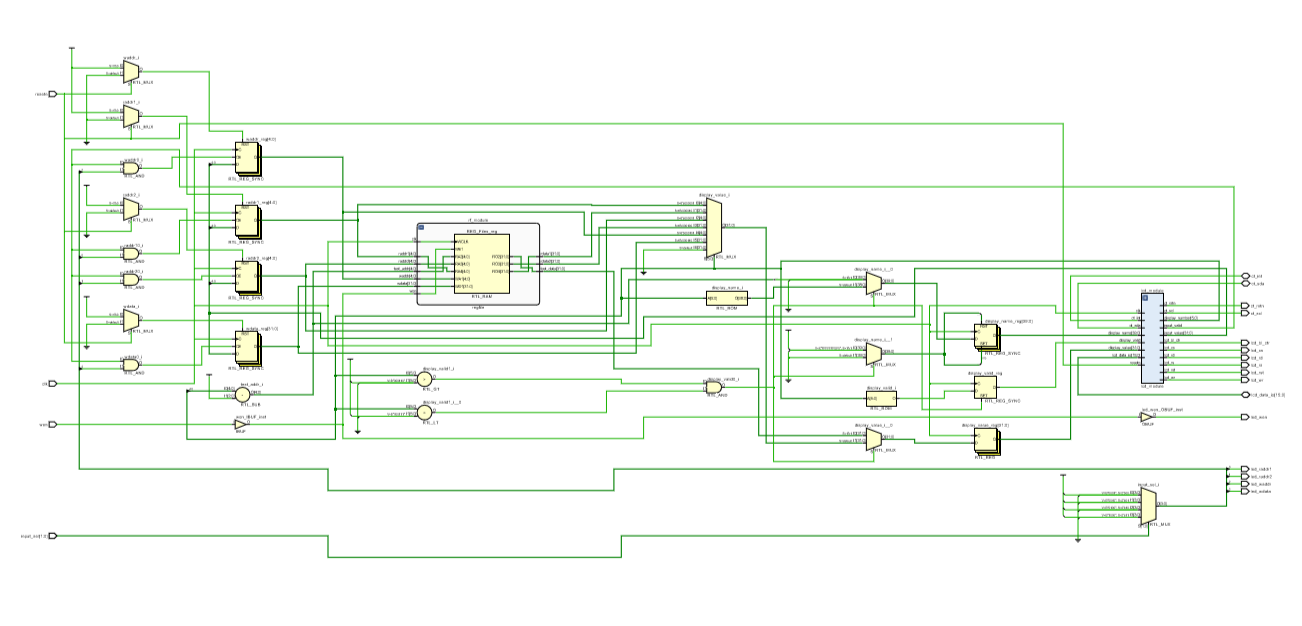最后附上本实验用到的所有文件：
可以使用百度云进行免费下载
链接：https://pan.baidu.com/s/17x4MJ9VBMOF8MhVe9dWKOA
提取码：itbo
如果本博客对你有所帮助，欢迎使用CSDN下载来支持我
链接：https://download.csdn.net/download/weixin_43074474/13728756


展开全文计算机组成原理实验 Verilog Vivado
• 需要用数组表示寄存器堆 寄存器堆需要有两个数据输出接口，同时输出；一个输入接口 0号寄存器不能保存数据，固定为0 读两个要求 1raddr1读地址 2raddr2 写四个要求 1waddr哪个寄存器 2wdata数据 3clk等脉冲上升沿 4W...
寄存器
寄存器是中央处理器内的组成部分
寄存器是有限存贮容量的高速存贮部件
用来暂存指令、数据和地址
寄存器堆
MIPS指令需要32个寄存器，采用寄存器-寄存器结构
需要用数组表示寄存器堆
寄存器堆需要有两个数据输出接口，同时输出；一个输入接口
0号寄存器不保存数据，固定为0【用来完成一些简单指令
读
raddr1读地址
若raddr=00111，则rdata1写入7号寄存器的数据
写四个要求
1 waddr哪个寄存器
2 wdata数据
3 clk等脉冲上升沿
4 WE读写信号高电平
寄存器堆接口
module registers（
input clk,
input oc,	//可不要
input [4:0] raddr1,
input [4:0] raddr2,
input [4:0] waddr,
input [31:0] wdata,
input we,	//使能端，读写信号
output reg[31:0] rdata1,
output reg[31:0] rdata2
);
reg [31:0] rdata1;
reg [31:0] rdata2;
reg [31:0] regts[1:31];
//读端口1读端口2用两个独立的always模块来实现
//读端口1
always @(*)	//组合逻辑电路，只要有输入马上就有输出
begin
if(oc==1'b1)	//禁止输出
begin
radata1<=32'b0;
end
else if(raddr1==5'b00000)	//$0号寄存器只保存0 begin rdata1<=32'b0; end else begin rdata1<=regts[raddr1] end end //读端口2 //写端口 always @（posedge clk) //脉冲信号作用下才能写 begin if((we==1'b1)&&(waddr!=5'b00000)) //判断使能端是否为1，是否为0号地址，0号不可写 begin regts[waddr]<=wdata; end end  ALU&&寄存器堆 寄存器堆rdata1接ALU a端口，寄存器堆rdata2接ALU b端口 ALU输出f端口接寄存器堆wdata端口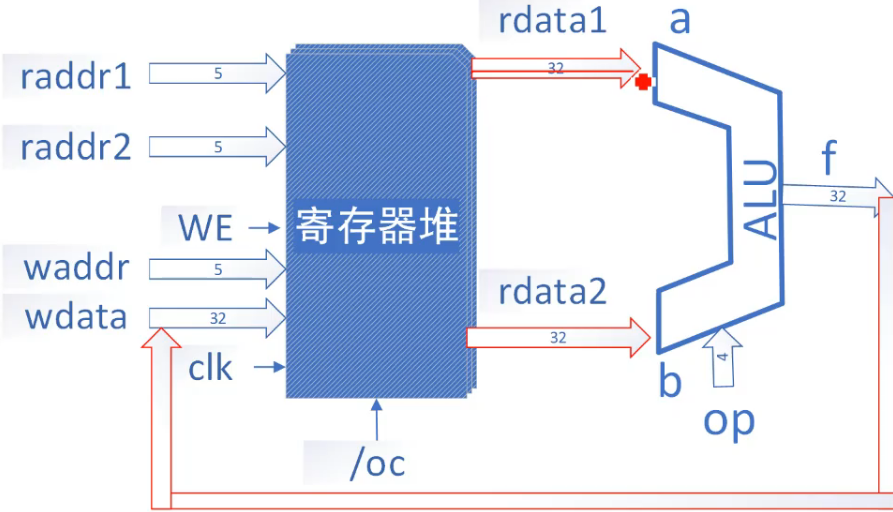reg(…out)输出端口与alu(a…)输入端口位数一致 wire[3:0]w, //声明wire电线连接，两两之间 reg r(…w), alu a(w…), fib整体框架图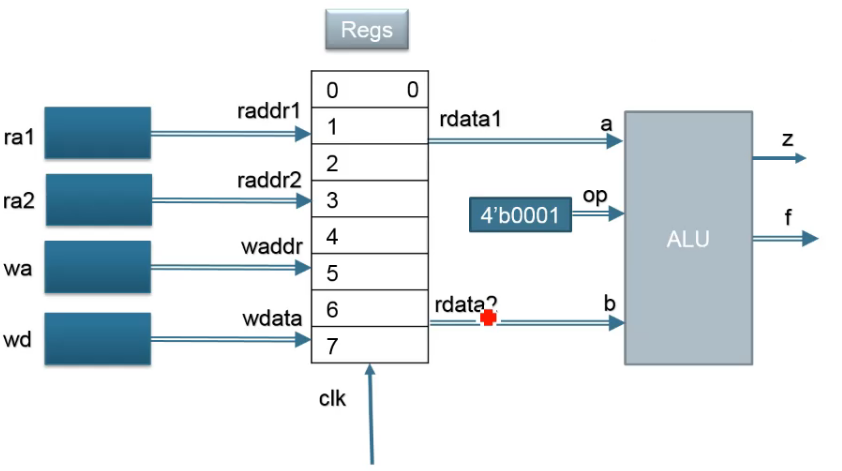操作确定：加法 默认：WE=1 初始除了0号寄存器其他寄存器均为空 通过写端口写入数据 rada1 rdata2数据来自寄存器 有限状态机fsm always@(posedge clk)//时钟脉冲的作用下 if(reset)//复位信号有效，直接跳转到初始状况 … else case(state) STATE1: … //指出每个状态执行完跳转到哪个状态 STATE1: … … default:state <= STATE1; endcase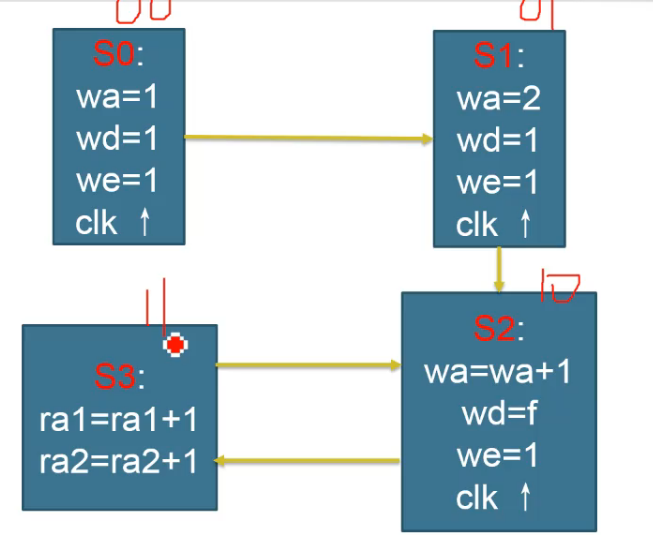fib&fsm //申请状态 wire [31:0] a, b ,f; reg [31:0] wdata; wire we = 1'b1; parameter op = 4'b0001; //加法运算 reg [4:0] ra1,ra2,wa; reg [3:0] count; //实例化寄存器 registers myregs(.clk(clk),.oc(~rst),.raddr1(ra1),.raddr2(ra2),.waddr(wa),.wda(wdata),.we(we),.rdata1(a),.rdata2(b)); //实例化ALU alu myalu(a, b, op, f); //a b与rdata1(a),rdata2(b)连接 assign result = wdata; reg[1:0]status = 2'b00; //状态 always@(posedge clk) begin if(rst == 1'b0) //复位信号 begin status <= 2'b00; //初始化为0 count <= 3; //从3开始算 end else case(status) 2’b00: begin ra1 <= 5'b1; ra2 <= 5'b10; wa <= 5'b1; //1号寄存器 wdata <= 32'b1; //1写入1号寄存器 status <= 2'b01; //跳转到下一状态 end 2’b01: begin wa <= 5'b10; wdata <= 32'b1; status <= 2'b10; end 2'b10: begin wa <= ra2+1; wdata <= f; status <= 2'b11; count <= count + 1; end 2'b11: begin if(count < n) begin ra1 <= ra2; //ra1=ra1+1 ra2 <= wa; //ra2=ra2+1 status <= 2'b10; end end endcase  最后补充-所有模块代码 //top模块： module top2( input clk, input rst, input [3:0] n, output [11:0] result ); wire [31:0] out; assign result=out[11:0]; fib1 f(clk,rst,n,out); initial$monitor($time,,"top:result=%h",result); endmodule // //fib模块： module fib1( input clk, input rst, input [3:0] n, output [31:0] result ); wire [31:0] a,b,f; reg [31:0] wdata; wire we = 1'b1; reg [4:0] ra1,ra2,wa; reg [3:0] count; parameter op = 4'b0001; //加法运算 //实例化寄存器 registers myregs(.clk(clk),.oc(~rst),.raddr1(ra1),.raddr2(ra2),.waddr(wa),.wdata(wdata),.we(we),.rdata1(a),.rdata2(b)); //实例化ALU alu myalu1(a, b, op, f); //a b与rdata1(a),rdata2(b)连接 assign result = wdata; reg[1:0]status = 2'b00; //状态 always@(posedge clk) begin if(rst == 1'b0) //复位信号 begin status <= 2'b00; //初始化为0 count <= 3; //从3开始算 end else case(status) 2'b00: begin ra1 <= 5'b1; ra2 <= 5'b10; wa <= 5'b1; //1号寄存器 wdata <= 32'b1; //1写入1号寄存器 status <= 2'b01; //跳转到下一状态 end 2'b01: begin wa <= 5'b10; wdata <= 32'b1; status <= 2'b10; end 2'b10: begin wa <= ra2+1; wdata <= f; status <= 2'b11; count <= count + 1; end 2'b11: begin if(count < n) begin ra1 <= ra2; //ra1=ra1+1 ra2 <= wa; //ra2=ra2+1 status <= 2'b10; end end endcase end initial$monitor($time,,"fib:rst=%b,wdata=%h,count=%h",rst,wdata,count); initial$monitor($time,,"fib:result=%h",result); endmodule // //alu模块： module alu( input [31:0] a, input [31:0] b, input [3:0] operation, output [31:0] f, output z ); reg[31:0]result; always@(*) begin case(operation) 4'b0000:result=32'b0; 4'b0001:result=a+b; 4'b0010:result=a-b; 4'b0011:result=a&b; 4'b0100:result=a|b; 4'b0101:result=a^b; default:result=32'b0; endcase end assign f=result; assign z=~(|result); initial$monitor($time,,"alu:a=%h,b=%h,operation=%b",a,b,operation); initial$monitor($time,,"alu:f=%h,z=%b",f,z); endmodule // //registers模块： module registers( input clk, input oc, //可不要 input [4:0] raddr1, input [4:0] raddr2, input [4:0] waddr, input [31:0] wdata, input we, //使能端，读写信号 output [31:0] rdata1, output [31:0] rdata2 ); reg [31:0] rdata1; reg [31:0] rdata2; reg [31:0] regts[1:31]; //读端口1 always @(*) //只要有输入就立即输出 begin if(oc == 1'b1) //禁止输出 begin rdata1 <= 32'b0; end else if(raddr1 == 5'b00000) //$0号寄存器只保存0
begin
rdata1 <= 32'b0;
end
begin
rdata1 <= regts[raddr1];
end
end
//读端口2
always @(*)
begin
if(oc == 1'b1)
begin
rdata2 <= 32'b0;
end
else if(raddr2 == 5'b00000)
begin
rdata2 <= 32'b0;
end
begin
rdata2 <= regts[raddr2];
end
end

always @(posedge clk)		//脉冲信号作用下才能写
begin
#1 if((we == 1'b1) &&(waddr != 5'b00000))		//判断使能端是否为1，是否为0号地址，0号寄存器不可写
begin
regts[waddr] <= wdata;
end
end
endmodule

//仿真程序
module fib_sim(
output [11:0] result
);
//parameter clk_period=10;
reg clk;
initial
begin
clk=1'b0;
forever
#2 clk=~clk;
end

reg rst=1'b1;
reg[3:0] n=4'b1111;
top2 t(clk,rst,n,result);

initial
begin
#2 rst=1'b0;
#4 rst=1'b1;
end

endmodule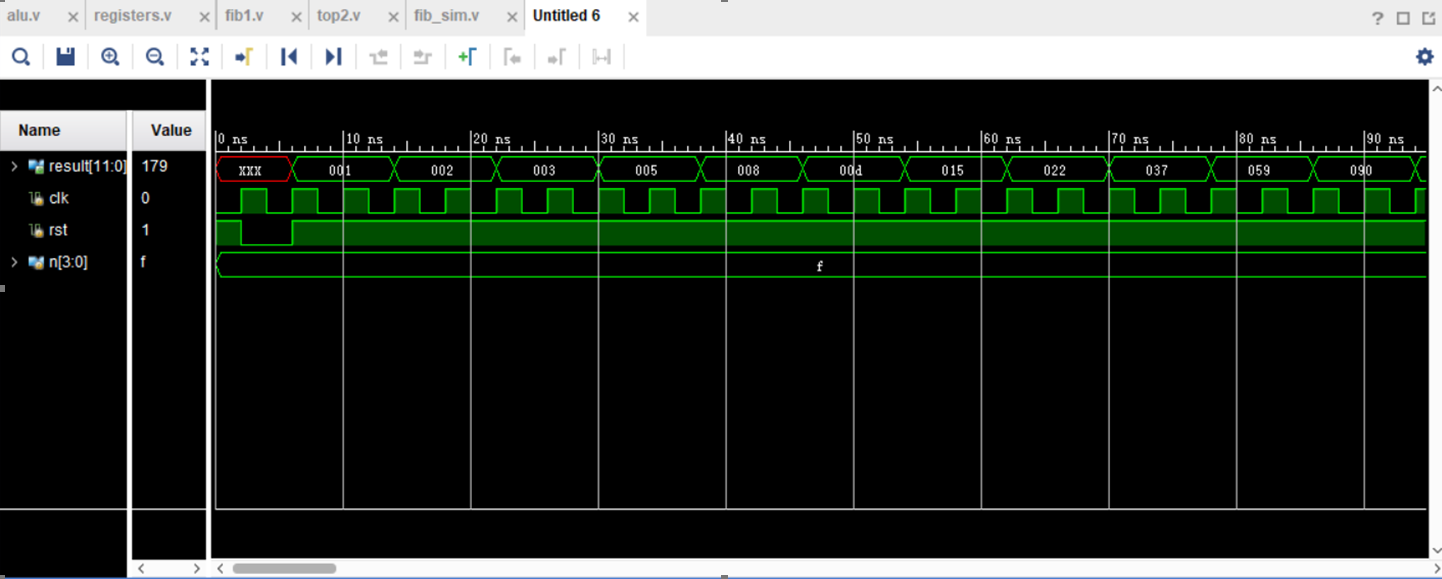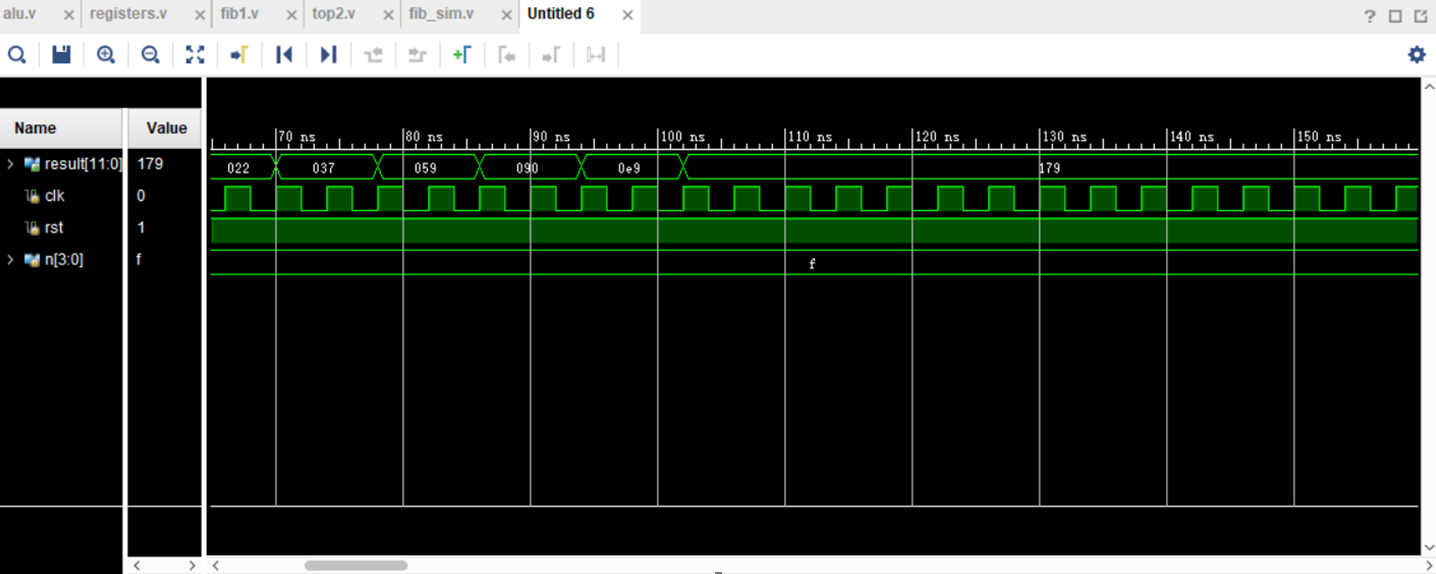仿真到179’h即停止的原因是输入n的位数为4位。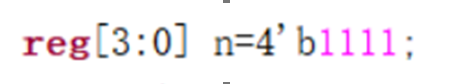展开全文fpga
• 3. 学习寄存器堆的数据传送与读/写工作原理，掌握寄存器堆的设计方法 解决方法 1. 分析一个32x32位的寄存器堆，即含有32个寄存器，每个寄存器32位。该寄存器堆有2个读端口、1个写端口，即能够同时读出2个寄存器的值...
实验内容
1.	学习使用Verilog HDL 进行时序电路的设计方法
2.	掌握灵活运用Verilog HDL进行各种描述与建模的技巧和方法
3.	学习寄存器堆的数据传送与读/写工作原理，掌握寄存器堆的设计方法
解决方法
1.	分析一个32x32位的寄存器堆，即含有32个寄存器，每个寄存器32位。该寄存器堆有2个读端口、1个写端口，即能够同时读出2个寄存器的值，写入1个寄存器
2.	首先，分析出两个端口读入是5位的地址，读出的数据是32位的两个，该寄存器堆只有一个写端口，还要配合控制信号Write_Reg，且写操作是边沿触发的，所有写入操作的输入信号必须在时钟边沿来临时已经有效
3.	寄存器堆的读操作和写操作的地址译码，只需引用寄存器地址作为数组的下标即可；读只需要组合逻辑，给出寄存器地址，读出数据
4.	代码展示：
顶层模块（需要数据输入在板子上操作）：
module RegisterFile(Addr,Write_Reg,Opt,Clk,Reset,A_B,LED);
input [1:0]Opt;
input [4:0]Addr;
input Write_Reg,Clk,Reset,A_B;
output reg [7:0]LED;
wire [31:0]R_Data_A,R_Data_B;
reg [4:0]R_Addr_A,R_Addr_B;
reg [31:0]W_Data;
initial
LED <= 0;
Fourth_experiment_first F1(R_Addr_A,R_Addr_B,Write_Reg,R_Data_A,R_Data_B,Reset,Clk,Addr,W_Data);
always@(Addr or Write_Reg or Opt or A_B or R_Data_A or R_Data_B)
begin
if(Write_Reg)
begin
case(Opt)
2'b00: begin W_Data=32'h000f_000f;  end
2'b01: begin W_Data=32'h0f0f_0f00;  end
2'b10: begin W_Data=32'hf0f0_f0f0;  end
2'b11: begin W_Data=32'hffff_ffff;  end
endcase
end
else
if(A_B)
begin
R_Addr_A=Addr;
case(Opt)
2'b00: LED=R_Data_A[7:0];
2'b01: LED=R_Data_A[15:8];
2'b10: LED=R_Data_A[23:16];
2'b11: LED=R_Data_A[31:24];
endcase
end
else
begin
R_Addr_B=Addr;
case(Opt)
2'b00: LED=R_Data_B[7:0];
2'b01: LED=R_Data_B[15:8];
2'b10: LED=R_Data_B[23:16];
2'b11: LED=R_Data_B[31:24];
endcase
end
end
endmodule


寄存器堆实现的Fourth_experiment_first模块
module Fourth_experiment_first(R_Addr_A,R_Addr_B,Write_Reg,R_Data_A,R_Data_B,Reset,Clk,W_Addr,W_Data);
input [4:0]R_Addr_A,R_Addr_B,W_Addr;
input Write_Reg,Reset,Clk;
input[31:0] W_Data;
output [31:0] R_Data_A,R_Data_B;
reg [31:0] REG_Files[0:31];
integer i=0;
always @ (posedge Clk or posedge Reset)
begin
if(Reset)
begin
for(i=0;i<=31;i=i+1)
REG_Files[i]<=0;
end
else
begin
if(Write_Reg)
REG_Files[W_Addr]<=W_Data;
end
end
assign R_Data_A = REG_Files[R_Addr_A];
assign R_Data_B = REG_Files[R_Addr_B];

endmodule

测试模块
module Fourth_experiment_test;
// Inputs
reg [4:0] Addr;
reg Write_Reg;
reg [1:0] Opt;
reg Clk;
reg Reset;
reg A_B;
// Outputs
wire [7:0] LED;
RegisterFile uut (
.Addr(Addr),
.Write_Reg(Write_Reg),
.Opt(Opt),
.Clk(Clk),
.Reset(Reset),
.A_B(A_B),
.LED(LED)
);
always #20 Clk = ~Clk;
initial begin
Addr = 5'b00001;
Write_Reg = 1;
Opt = 0;
Clk = 1;
Reset = 0;
A_B = 0;
#100;
Addr = 5'b00001;
Write_Reg = 0;
Opt = 0;
A_B = 0;
end
endmodule



展开全文• 寄存器文件（register file）又称寄存器堆，是CPU中多个寄存器组成的阵列，通常由快速的静态随机读写存储器（SRAM）实现。这种RAM具有专门的读端口与写端口，可以多路并发访问不同的寄存器。 CPU的指令集架构总是...
寄存器文件（register file）又称寄存器堆，是CPU中多个寄存器组成的阵列，通常由快速的静态随机读写存储器（SRAM）实现。这种RAM具有专门的读端口与写端口，可以多路并发访问不同的寄存器。

CPU的指令集架构总是定义了一批寄存器，用于在内存与CPU运算部件之间暂存数据。在更为简化的CPU，这些架构寄存器（architectural registers）一一对应与CPU内的物理存在的寄存器。在更为复杂的CPU，使用寄存器重命名技术，使得执行期间哪个架构寄存器对应于哪个寄存器堆的物理存储条目（physical entry stores）是动态改变的。寄存器堆是指令集架构的一部分，程序可以访问，这与透明的CPU高速缓存（cache）不同。
`
展开全文• 寄存器堆实验实验原理实验内容模块功能说明模块逻辑引脚图寄存器堆模块代码REG模块测试用例REG-ALU测试用例实验结果记录 寄存器堆实验结果及分析ALU_REG运算器模块实验结果及分析探索与思考 实验原理   32×32位...
• 实验内容与原理: CPU内部通常包含若干个通用寄存器，以暂存参加运算的数据和中间结果。寄存器速度快，个数少，但是RISC ... 所谓寄存器堆，就是一个寄存器的集合，为方便访问其中的寄存器，对寄存器堆中的寄存器进...计算机组成原理 ALU运算器
• 寄存器堆是CPU中多个寄存器组成的阵列，由32个32位的寄存器构成，两个读数据口(Ra->BusA Rb->BusB),一个写数据口(Rw ->BusW),写数据受使能信号Wen控制，在时钟的下降沿有效 第一种实现方法,代码如下 ...
• 设计一个32×32位的寄存器堆（即含有32个寄存器，每个寄存器32位）
• 在做寄存器堆的实验时，当给寄存器写入数值之后，显示屏上总是显示不出寄存器的内容，但是却可以输入该寄存器的地址，然后把寄存器的内容读出来。初步猜想是往寄存器里写内容应该是没问题的，因为可以通过寄存器的...Verilog
• 寄存器堆（register file）是CPU中多个寄存器组成的阵列，通常由快速的静态随机读写存储器（SRAM）实现。这种RAM具有专门的读端口与写端口，可以多路并发访问不同的寄存器。 本人所写的RF寄存器堆，其中包含4个8位...fpga
• 使用Logisim设计和实现mips寄存器堆（即，regFile），具体要求如下： 1，包含两个读寄存器号输入端口RD1和RD2，用来分别指定待读出数据的两个寄存器，这两个寄存器可能相同，也可能不同。读出的数据分别通过data1和...嵌入式 python 物联网 fpga 算法
• 实验二、CPU部件实现之ALU和寄存器堆 一、实验目的: 理解和掌握CPU中的算术逻辑运算部件(ALU)和寄存器堆(Register File)的工作 原理，并使用Verilog和ModelSim进行设计和仿真。 二、实验内容: 使用Verilog...
• 寄存器堆（Register File）是微处理的关键部件之一。寄存器堆往往具有多个读写端口，其中写端口往往与多个处理单元相对应。传统的方法是使用集中式寄存器堆，即一个集中式寄存器堆匹配N个处理单元。随着端口数量的...
• 写一写CPU中涉及到RAM的部件，如寄存器堆、数据存储器等。 　大家应该在大一刚接触到计算机的时候就知道ROM、RAM了吧。但也记不得那些繁杂的名称，只知道ROM是只读存储器，RAM是可读写存储器。其实知道这些也就够了...
• 实验内容 （1）自行设计寄存器堆实验的方案。 （2）根据设计的实验方案，编写相应代码。 （3）对编写的代码进行仿真，得到正确的波形图。 （4）将程序下载到实验箱运行、观察、修改。 2．基本要求 （1）熟悉并掌握......